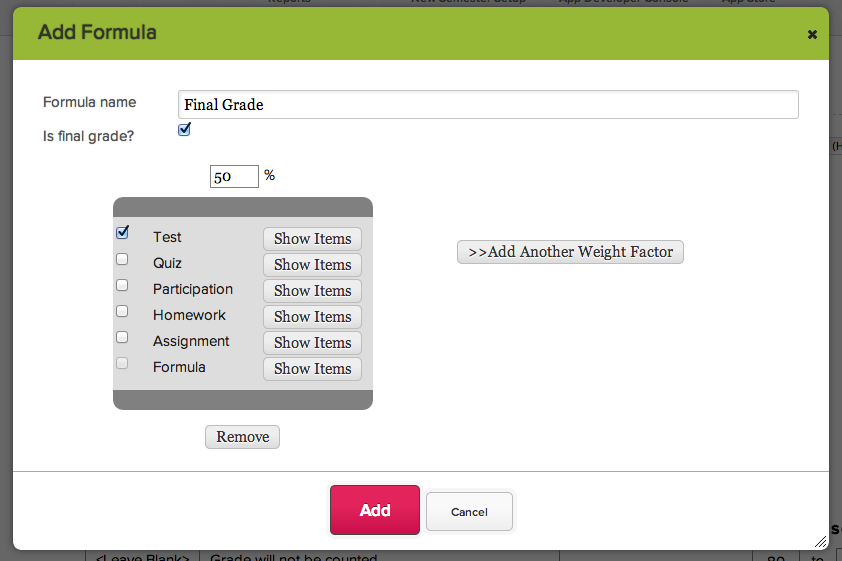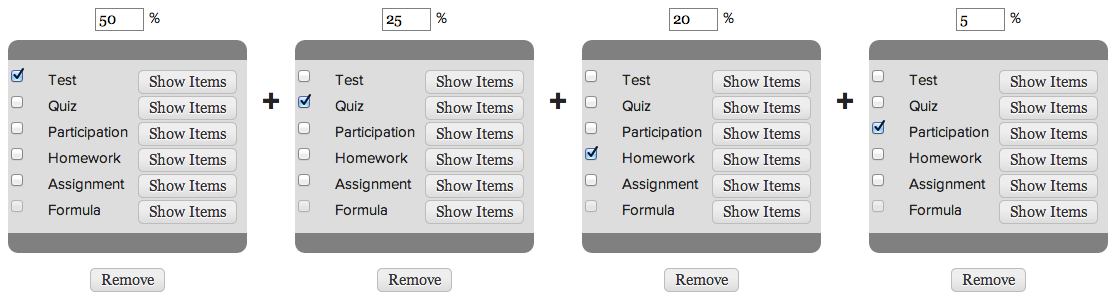This is a guest post by 2 of our awesome live chat agents: Anna and Rick

Many of our schools – and their teachers – prefer to use weighted grades when calculating final grades for a semester or for a course. If you are considering using weighted grades, or wondering how to get your grading all set up, here’s some info on how it all works.

Weighted grading is a grading system where certain types of assignments/assessments are prioritized more than others in the final grade. Often, the idea behind this sort of grading is smaller assignments are designed to be more like practice while tests and exams are designed to allow students really to show what they have learned. Therefore, these big grades ought to count for more in the final grade than practice grades should.

For many classes, weighted grades work in grading systems that rely on using graded assignments for feedback and calculating a student’s final grade. This final grade is a weighted average. This sort of average is designed to make the final grade be closest to the average of the type of assignment that is the most heavily weighted.

Here’s an example of how weighted averages might be used. Say you are a History teacher, and you give 3 big tests per term, a dozen quizzes, homework everyday, and a daily participation grade. However, you want these three big tests to be the biggest portion of a student’s grade because they require a student to demonstrate their best writing and all of their knowledge to date. You might decided that for the grading system in your class, tests will be 50%, quizzes will be 25%, homework will be 20%, and participation will be 5%. As a result in this class, tests have by far the largest impact on a student’s final grade, quizzes have half of that impact, homework has a little less, and participation has even less of an impact.  At the end of term, the way students’ final averages would be calculated following a formula that looks a lot like this :

Final Grade = (sum of tests’ averages) * 0.5 + (sum of quizzes’ averages)*0.25 + (sum of homeworks’ averages) * 0.20 + (sum of participation averages) * 0.05

Looks like a lot of time with a calculator, right? Well, that’s where our Gradebook enters the game.

## Setting Up Formulas in Gradebook

Sounds great, right? Well, here’s how to set it up for any Gradebook.

1 – In a teacher’s gradebook, click the green + Add Formula button. A window will open up allowing you to set up the formula.

2 – Enter a weight percentage for a category in the text field over the grey category box. So, if you were that History teacher who wanted tests to be 50% of the final grade, you would write “50”. Then, check the category that has this weight percentage – tests.3 – Continue to add weighted percentages for your categories until your percentages equal 100%. Use the >> Add Weight Factor button to continue to add categories and their weight percents. A complete formula might look something like our History class formula, shown below.This way of setting up weighted final grades will work for any formula you may need to set up in Gradebook. It’s a powerful tool for customizing the way graded feedback will work in a class. Of course, if you need any help setting up weighted final grades or other formulas, please do chat in.